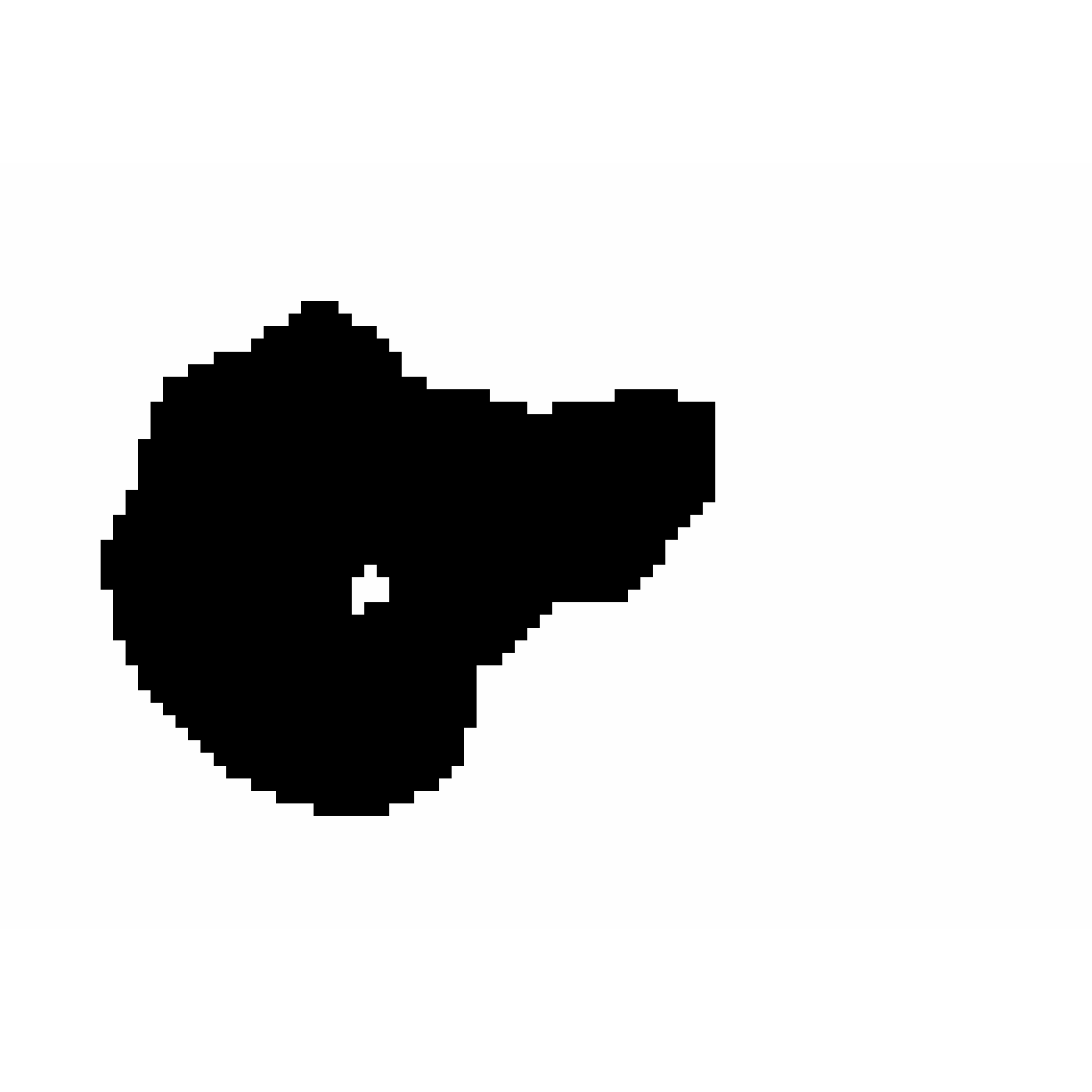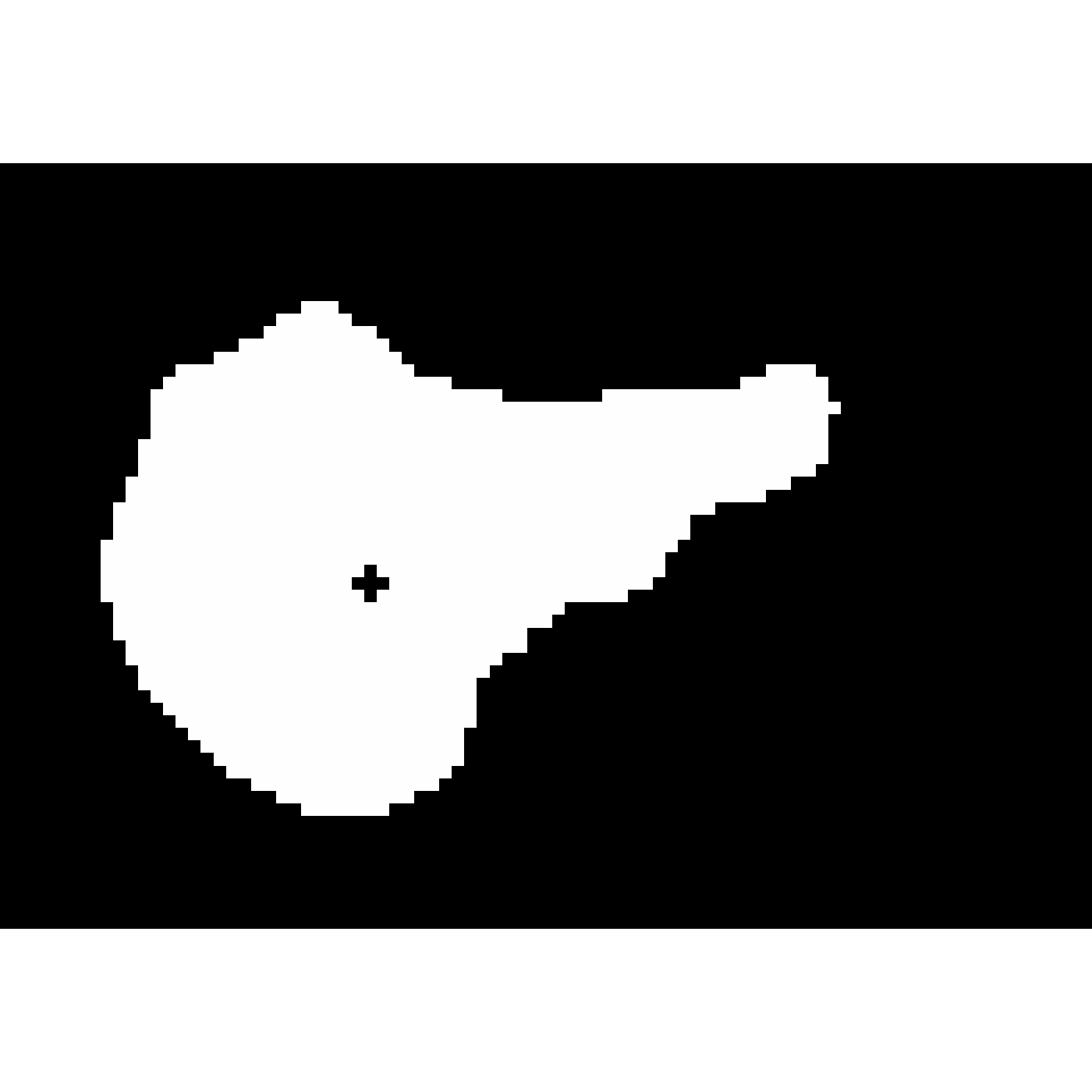Takes an matrix (or and returns the nearest distance to each TRUE.

render_boolean_distance(boolean, rescale = FALSE)

## Arguments

boolean

Logical matrix (or matrix of 1s and 0s), where distance will be measured to the TRUE values.

rescale

Default FALSE. Rescales the calculated distance to a range of 0-1. Useful for visualizing the distance matrix.

## Value

Matrix of distance values.

## Examples

#if(interactive()){
#Measure distance to
image(render_boolean_distance(volcano > 150))image(render_boolean_distance(volcano < 150))#If we want to rescale this to zero to one (to visualize like an image), set rescale=TRUE
plot_image(render_boolean_distance(volcano > 150,rescale=TRUE))
#> Error in plot_image(render_boolean_distance(volcano > 150, rescale = TRUE)): object 'xlim' not found
#end}